# [CVPR 2019论文阅读笔记]Self-Supervised Learning of 3D Human Pose using Multi-view Geometry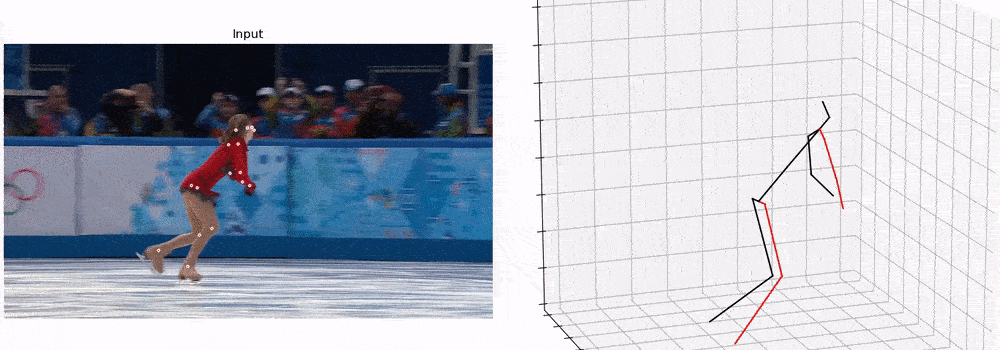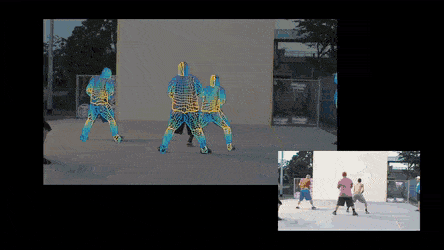（1）3D pose from 2D keypoint detection:

• 先从图像空间(image space)中检测二维关键点（2D keypoints）
• 再从2D 提升到 (lift to) 3D，这一步还是比较简单的，用一个简单的神经网络就能学习2D 到3D 的表示

（2）Direct 3D pose estimation

#### 主要方法–训练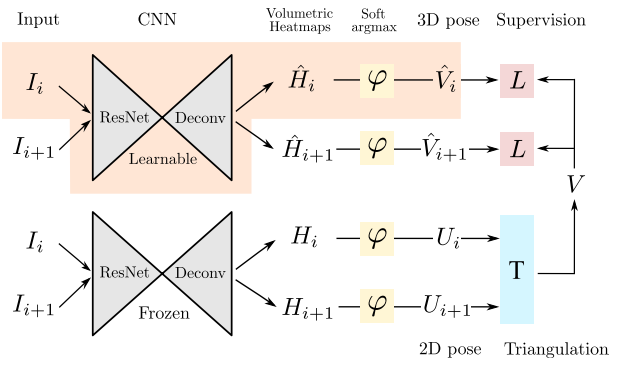$\left[ \begin{matrix} x_{i, j} \\ y_{i, j} \\ w_{i, j} \end{matrix} \right] = K[R|RT] \left[ \begin{matrix} X_j \\ Y_j \\ Z_j \\ 1 \end{matrix} \right]$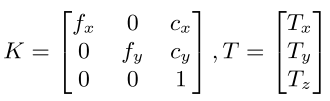K代表相机内参，R是旋转矩阵，T是平移矩阵。

#### Inference推理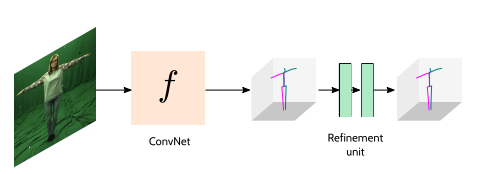#### Pose Structure Score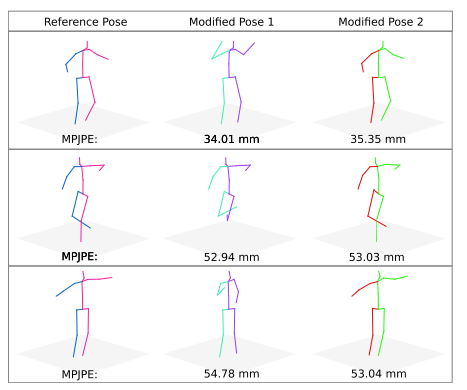##### 如何计算PSS？

$PSS(p, q) = \delta(C(p), C(q)) \\ C(p) = \mathop{\arg\min}_k||p-\mu_k||_2^2\\ \delta(i, j)=\begin{cases}1,& i = j \\ 0,& i \neq j \end{cases}$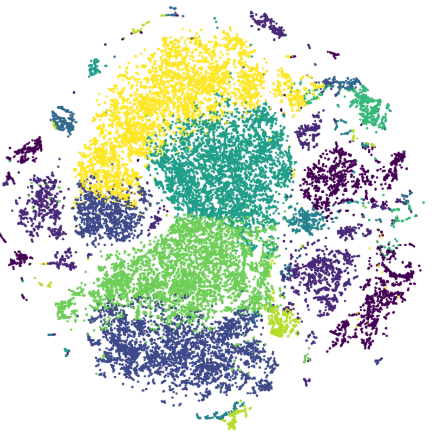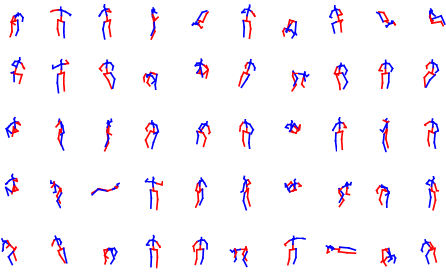#### 实验结果

• 数据库: Human 3.6M, MPI-INF-3DHP
• Metrics: MPJPE, PCK, PSS
##### Can we rely on the labels from multi view images?

GT表示用H36M中的2D标签，GT 2D (w/o R)表示不用camera geometry(旋转矩阵R) H36M 2D 和MPII 2D表示在这些数据集上训练的姿态估计模型。我们可以看到，用GT 2D的效果最好，其次是H36M 2D，而在MPII数据集上训练的2D keypoints 检测器有轻微的效果下降。作者认为是因为H36M中的数据是用marker得到的，更准确(H36M中数据集中的试验者在身上贴有小亮片（传感器)）。而MPII中人的关键点是由人工标记的，一些关键点定位不准。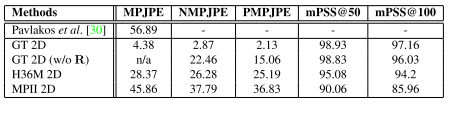##### Comparison to State-of-the-art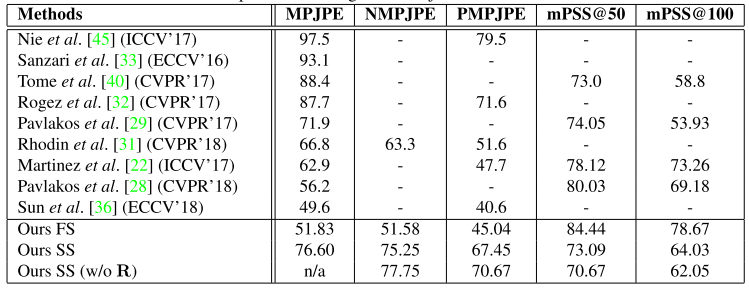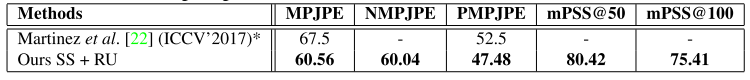EpipolarPose在H36M上最好的结果能达到60.56mm(MPJPE)。

##### Weakly/Self Supervised Methods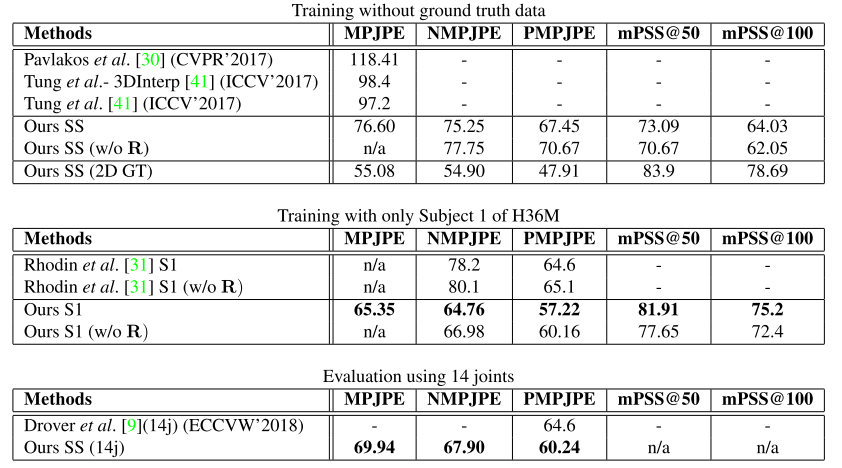##### 定性结果展示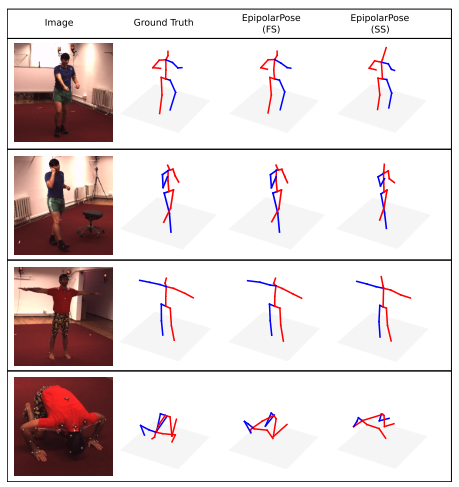#### 思考©️2019 CSDN 皮肤主题: 书香水墨 设计师: CSDN官方博客